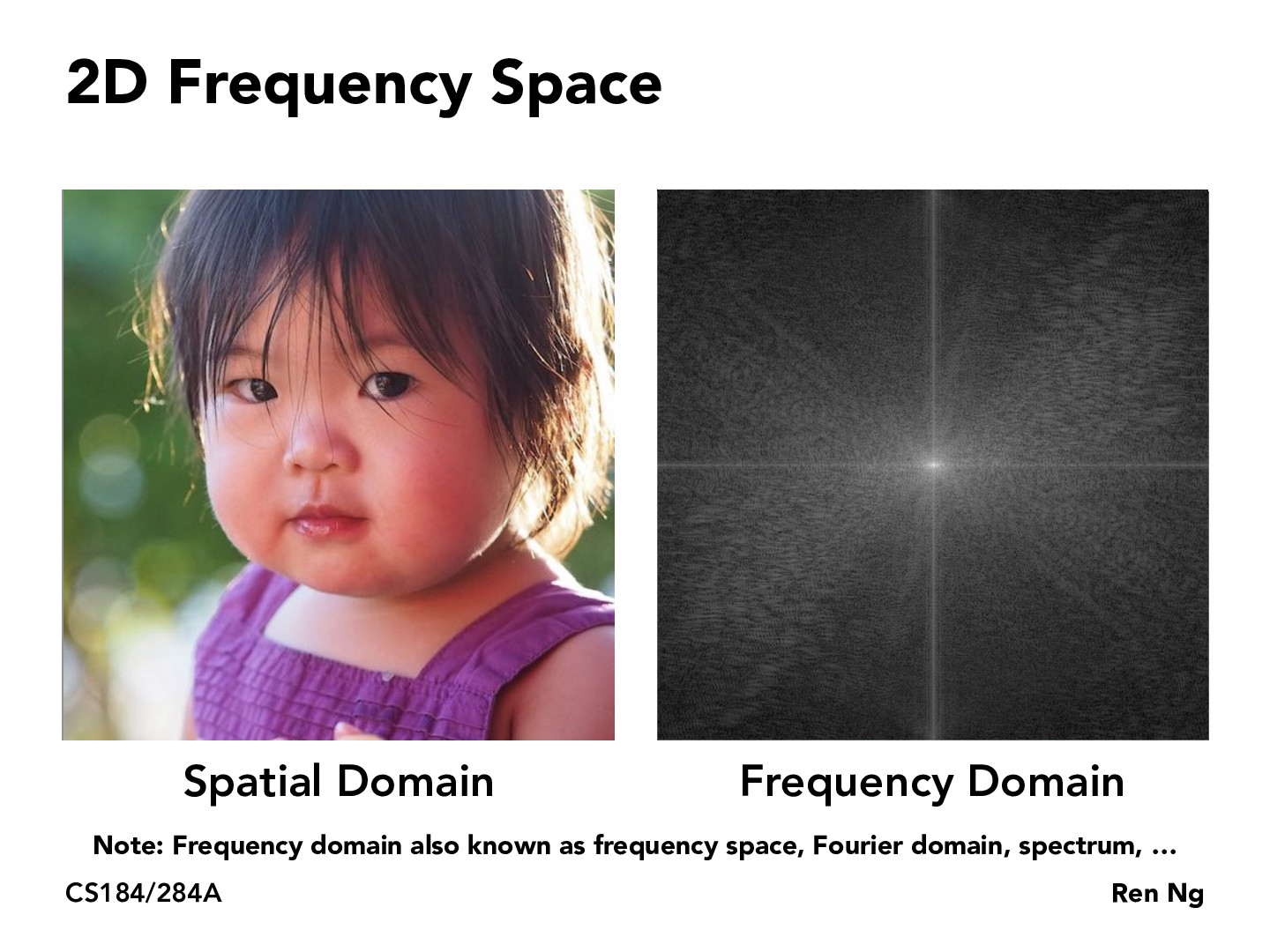You are viewing the course site for a past offering of this course. The current offering may be found here.Lecture 3: Sampling, Aliasing, Antialiasing (33)AidanJDeAngelis

Are all frequency diagrams symmetric about the origin because the sine function is odd?ivyn

In the context of image processing, Fourier transforms decompose an image into its sine and cosine components. The input image is the spatial domain, a space where distances in pixels directly correspond to real distances (e.g. meters). The output of the transformation represents the image in the frequency domain, a space where an image value at an image position represents the amount that the intensity values in the image vary over a specific distance related to that position.

https://homepages.inf.ed.ac.uk/rbf/HIPR2/fourier.htmkatamarisun

To @Aidan's question, an interesting property is that the Fourier transform for all real signals (including photos) is conjugate symmetric about the axis, meaning X(jw) = X*(-jw). When plotting the frequency domain, generally the magnitude is plotted, and for any value, |x^2| = |(x*)^2|, which is why there's this nice symmetry in the frequency domain. Specifically, any straight line through the origin will be symmetric / even!

You must be enrolled in the course to comment